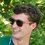# Do you know any formulas to evaluate sums of series?

State MATHCOUNTS are coming up, and I really need to find a good method for finding sums of series and sequences. I have scoured the internet about this, and I can't find a single method! It would be great if you guys could post an explanation to solve a problem say, like this: $\sum_{x=1}^{45} 1/2x$. Also, what is the difference between a series and a sequence? Are there formulas for geometric and arithmetic sequences/series? How can you evaluate an infinite converging series/sequence? I'm totally clueless, and any clues would be unbelievably helpful!Note by Finn Hulse
7 years, 5 months ago

This discussion board is a place to discuss our Daily Challenges and the math and science related to those challenges. Explanations are more than just a solution — they should explain the steps and thinking strategies that you used to obtain the solution. Comments should further the discussion of math and science.

When posting on Brilliant:

• Use the emojis to react to an explanation, whether you're congratulating a job well done , or just really confused .
• Ask specific questions about the challenge or the steps in somebody's explanation. Well-posed questions can add a lot to the discussion, but posting "I don't understand!" doesn't help anyone.
• Try to contribute something new to the discussion, whether it is an extension, generalization or other idea related to the challenge.

MarkdownAppears as
*italics* or _italics_ italics
**bold** or __bold__ bold
- bulleted- list
• bulleted
• list
1. numbered2. list
1. numbered
2. list
Note: you must add a full line of space before and after lists for them to show up correctly
paragraph 1paragraph 2

paragraph 1

paragraph 2

[example link](https://brilliant.org)example link
> This is a quote
This is a quote
    # I indented these lines
# 4 spaces, and now they show
# up as a code block.

print "hello world"
# I indented these lines
# 4 spaces, and now they show
# up as a code block.

print "hello world"
MathAppears as
Remember to wrap math in $$ ... $$ or $ ... $ to ensure proper formatting.
2 \times 3 $2 \times 3$
2^{34} $2^{34}$
a_{i-1} $a_{i-1}$
\frac{2}{3} $\frac{2}{3}$
\sqrt{2} $\sqrt{2}$
\sum_{i=1}^3 $\sum_{i=1}^3$
\sin \theta $\sin \theta$
\boxed{123} $\boxed{123}$

Sort by:

I have trouble understanding series, too.

However, from my understanding, I have a few ideas in mind (credibility = basically 0):

$\rightarrow$ I THINK (please correct me if I'm wrong :D) the sum of an infinite geometric series is $\frac{a}{1-r},$ where $a$ represents the first term and $r$ represents the constant ratio...

$\rightarrow$ I THINK (please correct me if I'm wrong :D) that the summation that you posted above is means that there are 45 terms, and you are asked to find the sum of the series in which the first term is $\frac{1}{2}$, and all other terms follow the sequence $\frac{1}{2}, \frac{1}{2\times2}, \frac{1}{2\times3}, \frac{1}{2\times4}, ..., \frac{1}{2\times45},$ though I have no idea how to sum that. (Telescoping?)

Hope this helps, and I hope to learn more about these convoluted series and sums with you!

- 7 years, 5 months ago

Awesome! That's actually really cool! Isn't it so weird that there seems to be nothing about these kind of formulas, like, anywhere? These kind of problems pop up ALL the time, but I always have to find some wacky way to solve them. Thanks for the infinite geometric progression formula!

- 7 years, 5 months ago

Yeah no problem :D, I have trouble with some MathCounts concepts too. I still have a lot to learn :D

On a side note, good luck on MathCounts States! (I'm going for MD)

- 7 years, 5 months ago

I'm going for VA! Maybe I'll see you at Nationals! (Probably not if I can't nail this series stuff ;)).

- 7 years, 5 months ago

I probably won't make Nats :/

Mr. Thomas Luo and the Takoma Park people (cough Will Cui cough) are too overpowered ._.

- 7 years, 5 months ago

Haha.

- 7 years, 5 months ago

Wait! Will Cui! I just saw a bunch of his solutions on AoPS! Right after you said that! That's hilarious!

- 7 years, 5 months ago

May I ask how you have Level 5 ratings if you can barely recall the geometric series formula?

- 7 years, 5 months ago

Sorry if I offended you in some way, but ratings and levels do not necessarily indicate how good you truthfully are in a subject. For example, the diagnostic problem for me may have been easier than other problems of the same level, or I may have a deeper understanding of some topics in a subject more than others.

If I don't recall the geometric series formula, that simply means that I am incompetent. I may not deserve my level or rating.

- 7 years, 5 months ago

It shows that he's awesome at solving problems, not at remembering formulas.

- 7 years, 5 months ago

Hi Finn!

There isn't much difference between a sequence and a series. Informally speaking, a sequence is a bunch of numbers, and a series is the sum of the individual terms. There are formulae to calculate the sum of terms of an arithmetic progression, a geometric progression, harmonic progression and arithmetico-geometrico progression. I think you should easily be able to find a good website to explain these. Hope this helps a little.

- 7 years, 5 months ago

Could you give me a link? I mean, I'd really just like to know how to sum arithmetic progressions.

- 7 years, 5 months ago

I found this quite useful when I learnt it, since it has useful related links on the sidebar too. But if it doesn't serve your purpose, you could always try Wolfram MathWorld or Wikipedia.

- 7 years, 5 months ago

Oh!!! I get it now! Thanks!

- 7 years, 5 months ago

I know to solve recurrences.Also have formulae for that.

- 7 years, 5 months ago

What are those? What's the formula?

- 7 years, 5 months ago